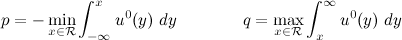#basque center for applied mathematics

You are in:

1. Research
2. Areas
3. Analysis of PDEs
4. Large-time behavior of numerical schemes for 1D scalar conser...

## Partial differential Equations, Numerics and Control

### Large-time behavior of numerical schemes for 1D scalar conservation laws

Alejandro Pozo

It is well known that the large-time asymptotic profile of 1D scalar conservation laws with strictly convex flux is the so-called N-wave, which depends on two parameters, p and q, given byThis behavior is lost whenever the solution is approximated by vanishing viscosity, since the asymptotic profile of this only depends on the mass of the initial data. Therefore, it is either completely positive or completely negative, in contrast to the pure hyperbolic case.

As 3-point monotone schemes add artificial viscosity to the solution, this lack of conmutativiy will also appear, presumably, at the discrete level. In the video presented above we show that the Lax-Friedrichs scheme happens to develop this pathology.

Nevertheless, this performance is not a general issue and there are schemes (for instance, Engquist-Osher and Godunov numerical fluxes) that lose the viscosity as time tends to infinity, capturing the N-wave properly.

Reference:
Ignat L.I., Pozo A., Zuazua E., Large-time asymptotics, vanishing viscosity and numerics for 1-D scalar conservation laws. Mathematics of Computation, to appear (preprint)

Back to list